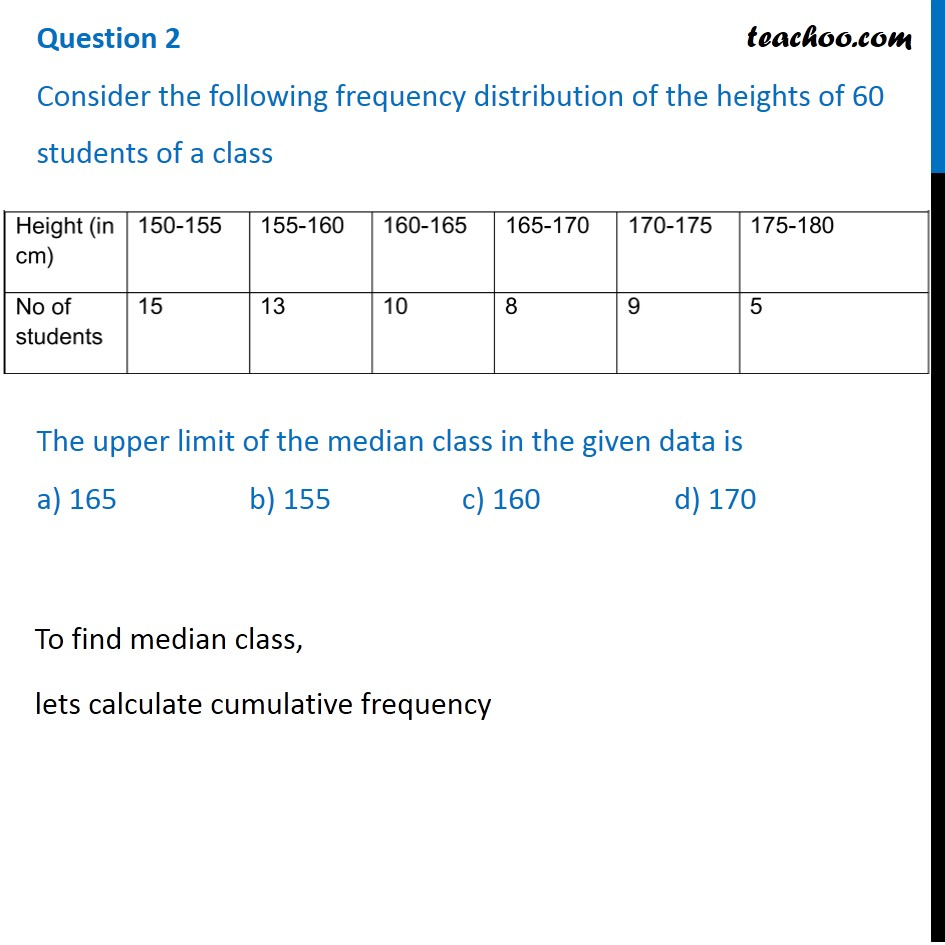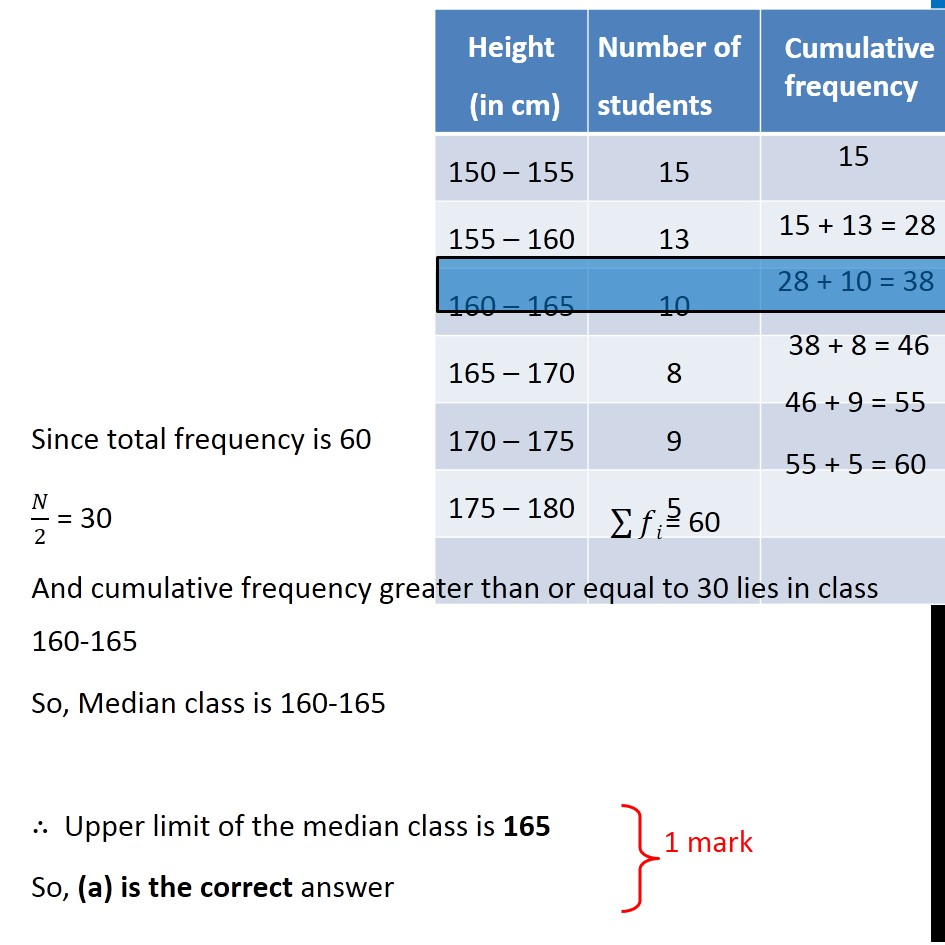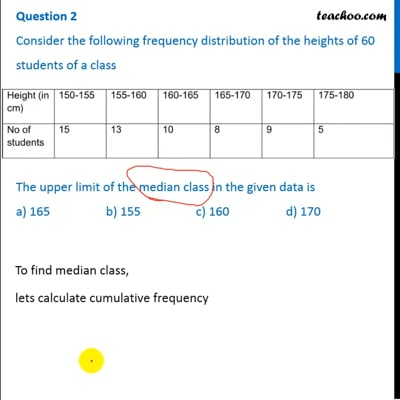CBSE Class 10 Sample Paper for 2020 Boards - Maths Standard

Class 10
Solutions of Sample Papers for Class 10 Boards

## Consider the following frequency distribution of the heights of 60 students of a class

 Height 150-155 155-160 160-165 165-170 170-175 175-180 No Of Students 15 13 10 8 9 5

## a) 165             b) 155             c) 160           d) 170This video is only available for Teachoo black users

### Transcript

Question 2 Consider the following frequency distribution of the heights of 60 students of a class The upper limit of the median class in the given data is a) 165 b) 155 c) 160 d) 170 To find median class, lets calculate cumulative frequency Height Number of Cumulative frequency (in cm) students 150 – 155 15 15 155 – 160 13 15 + 13 = 28 160 – 165 10 28 + 10 = 38 165 – 170 8 38 + 8 = 46 170 – 175 9 46 + 9 = 55 175 – 180 5 55 + 5 = 60 Since total frequency is 60 𝑁/2 = 30 And cumulative frequency greater than or equal to 30 lies in class 160-165 So, Median class is 160-165 ∴ Upper limit of the median class is 165 So, (a) is the correct answer